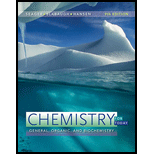Chapter 9, Problem 9.58E### Chemistry for Today: General, Orga...

9th Edition
Spencer L. Seager + 2 others
ISBN: 9781305960060

#### Solutions

Chapter
Section### Chemistry for Today: General, Orga...

9th Edition
Spencer L. Seager + 2 others
ISBN: 9781305960060
Textbook Problem

# Write balanced molecular, total ionic, and net ionic equations to represents neutralization reactions between RbOH and the following acids. Use all H's possible for each acid.a. H 3 PO 4 b. H 2 C 2 O 4 (oxalic acid)c. HC 2 H 3 O 2

Interpretation Introduction

(a)

Interpretation:

The balanced molecular, total ionic and net ionic form of the given reaction is to be stated.

Concept introduction:

In a balanced molecular chemical equation, all the reactants and products are written with their stoichiometric coefficient and physical states. The total ionic equation is the chemical equation of a reaction in which the ionic compounds are written in their dissociated ionic forms. The net ionic equation is the one in which the common ions present on both the sides of the complete ionic reaction get eliminated and only the chemical species actually participating in the reaction is represented.

Explanation

The base RbOH will react with H3PO4 to form Rb3PO4 salt and water. The balanced molecular equation for the given reaction is represented as,

3RbOH(aq)+H3PO4(aq)Rb3PO4(aq)+3H2O(l)

The total ionic chemical equation of the given reaction is represented as,

3Rb+(aq)+3OH(aq)+3H+(aq)+PO43(aq)3

Interpretation Introduction

(b)

Interpretation:

The balanced molecular, total ionic and net ionic form of the given reaction is to be stated.

Concept introduction:

In a balanced molecular chemical equation, all the reactants and products are written with their stoichiometric coefficient and physical states. The total ionic equation is the chemical equation of a reaction in which the ionic compounds are written in their dissociated ionic forms. The net ionic equation is the one in which the common ions present on both the sides of the complete ionic reaction get eliminated and only the chemical species actually participating in the reaction is represented.

Interpretation Introduction

(c)

Interpretation:

The balanced molecular, total ionic and net ionic form of the given reaction is to be stated.

Concept introduction:

In a balanced molecular chemical equation, all the reactants and products are written with their stoichiometric coefficient and physical states. The total ionic equation is the chemical equation of a reaction in which the ionic compounds are written in their dissociated ionic forms. The net ionic equation is the one in which the common ions present on both the sides of the complete ionic reaction get eliminated and only the chemical species actually participating in the reaction is represented.

### Still sussing out bartleby?

Check out a sample textbook solution.

See a sample solution

#### The Solution to Your Study Problems

Bartleby provides explanations to thousands of textbook problems written by our experts, many with advanced degrees!

Get Started

## Additional Science Solutions

#### Find more solutions based on key concepts